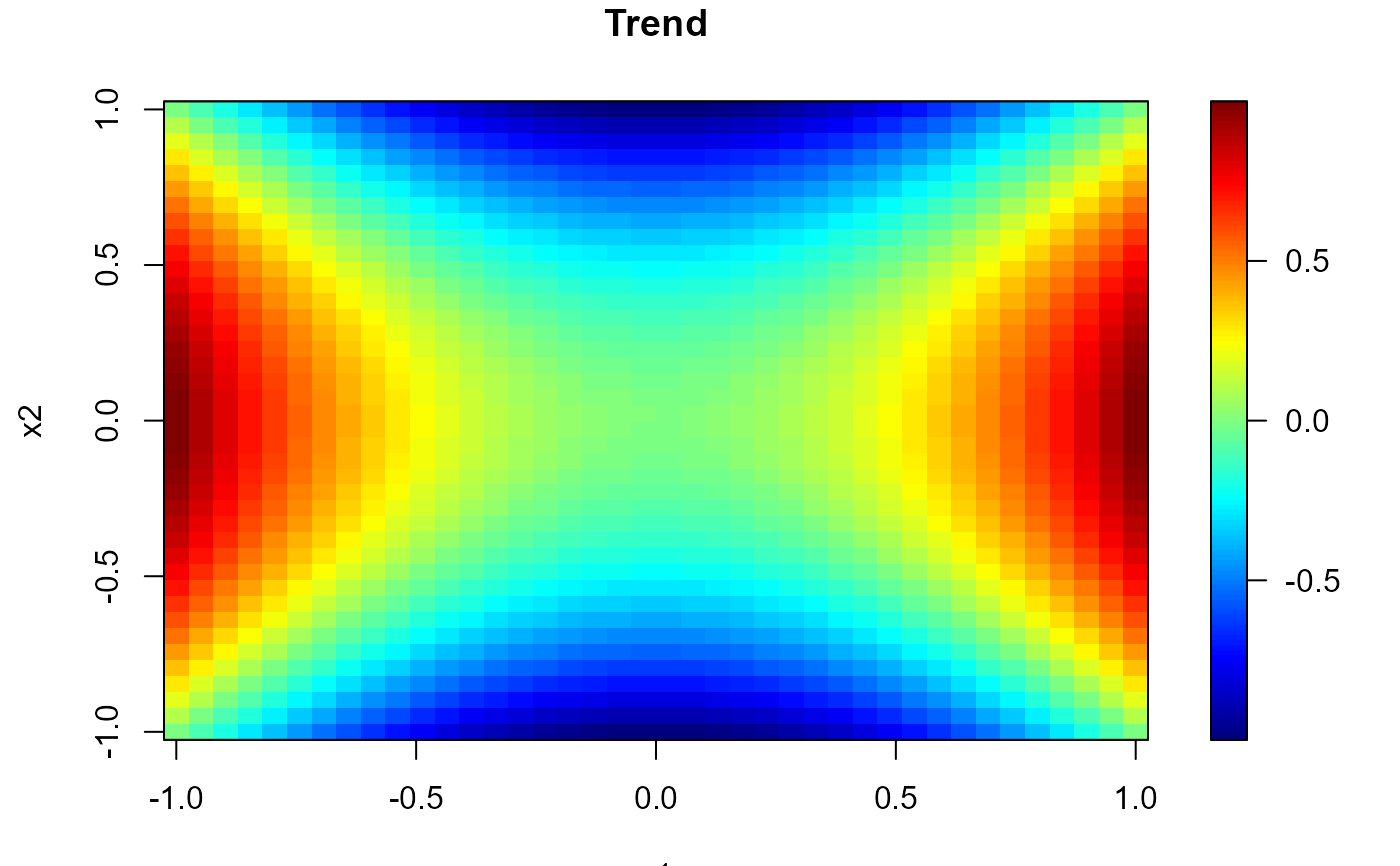simage (generic function) draws an image (a grid of colored rectangles) and (optionally) adds a legend strip with the color scale (calls splot and image).

plot.np.den calls simage.data.grid (contour and points also by default).

simage(x, ...)

# S3 method for default
simage(
x = seq(0, 1, len = nrow(s)),
y = seq(0, 1, len = ncol(s)),
s,
slim = range(s, finite = TRUE),
col = jet.colors(128),
breaks = NULL,
legend = TRUE,
horizontal = FALSE,
legend.shrink = 1,
legend.width = 1.2,
legend.mar = ifelse(horizontal, 3.1, 5.1),
legend.lab = NULL,
bigplot = NULL,
smallplot = NULL,
lab.breaks = NULL,
axis.args = NULL,
legend.args = NULL,
graphics.reset = FALSE,
xlab = NULL,
ylab = NULL,
asp = NA,
...
)

# S3 method for data.grid
simage(x, data.ind = 1, xlab = NULL, ylab = NULL, ...)

# S3 method for np.den
plot(
x,
y = NULL,
log = TRUE,
contour = TRUE,
points = TRUE,
col = hot.colors(128),
tolerance = npsp.tolerance(),
...
)

## Arguments

x grid values for x coordinate. If x is a list, its components x$x and x$y are used for x and y, respectively. For compatibility with image, if the list has component z this is used for s. additional graphical parameters (to be passed to image or simage.default; e.g. xlim, ylim, ...). NOTE: graphical arguments passed here will only have impact on the main plot. To change the graphical defaults for the legend use the par function beforehand (e.g. par(cex.lab = 2) to increase colorbar labels). grid values for y coordinate. matrix containing the values to be used for coloring the rectangles (NAs are allowed). Note that x can be used instead of s for convenience. limits used to set up the color scale. color table used to set up the color scale (see image for details). (optional) numeric vector with the breakpoints for the color scale: must have one more breakpoint than col and be in increasing order. logical; if TRUE (default), the plotting region is splitted into two parts, drawing the image plot in one and the legend with the color scale in the other. If FALSE only the image plot is drawn and the arguments related to the legend are ignored (splot is not called). logical; if FALSE (default) legend will be a vertical strip on the right side. If TRUE the legend strip will be along the bottom. amount to shrink the size of legend relative to the full height or width of the plot. width in characters of the legend strip. Default is 1.2, a little bigger that the width of a character. width in characters of legend margin that has the axis. Default is 5.1 for a vertical legend and 3.1 for a horizontal legend. label for the axis of the color legend. Default is no label as this is usual evident from the plot title. plot coordinates for main plot. If not passed these will be determined within the function. plot coordinates for legend strip. If not passed these will be determined within the function. if breaks are supplied these are text string labels to put at each break value. This is intended to label axis on a transformed scale such as logs. additional arguments for the axis function used to create the legend axis (see image.plot for details). arguments for a complete specification of the legend label. This is in the form of list and is just passed to the mtext function. Usually this will not be needed (see image.plot for details). logical; if FALSE (default) the plotting region (par("plt")) will not be reset to make it possible to add more features to the plot (e.g. using functions such as points or lines). If TRUE will reset plot parameters to the values before entering the function. label for the x axis, defaults to a description of x. label for the y axis, defaults to a description of y. the y/x aspect ratio, see plot.window. integer (or character) with the index (or name) of the component containing the values to be used for coloring the rectangles. logical; if TRUE (default), log(x$est) is ploted. logical; if TRUE (default), contour lines are added. logical; if TRUE (default), points at x$data\$x are drawn. tolerance value (lower values are masked).

## Value

Invisibly returns a list with the following 3 components:

bigplot

plot coordinates of the main plot. These values may be useful for drawing a plot without the legend that is the same size as the plots with legends.

smallplot

plot coordinates of the secondary plot (legend strip).

old.par

previous graphical parameters (par(old.par) will reset plot parameters to the values before entering the function).

## Side Effects

After exiting, the plotting region may be changed (par("plt")) to make it possible to add more features to the plot (set graphics.reset = FALSE to avoid this).

splot, spoints, spersp, image, image.plot, data.grid.

## Author

Based on image.plot function from package fields: fields, Tools for spatial data. Copyright 2004-2013, Institute for Mathematics Applied Geosciences. University Corporation for Atmospheric Research.

Modified by Ruben Fernandez-Casal <rubenfcasal@gmail.com>.

## Examples


#
# Regularly spaced 2D data
nx <- c(40, 40) # ndata =  prod(nx)
x1 <- seq(-1, 1, length.out = nx)
x2 <- seq(-1, 1, length.out = nx)
trend <- outer(x1, x2, function(x,y) x^2 - y^2)
simage( x1, x2, trend, main = 'Trend')#
# Multiple plots
set.seed(1)
y <- trend + rnorm(prod(nx), 0, 0.1)
x <- as.matrix(expand.grid(x1 = x1, x2 = x2)) # two-dimensional grid
# local polynomial kernel regression
lp <- locpol(x, y, nbin = nx, h =  diag(c(0.3, 0.3)))
# 1x2 plot
old.par <- par(mfrow = c(1,2))
simage( x1, x2, y, main = 'Data')
simage(lp, main = 'Estimated trend')par(old.par)